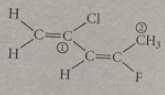# Problem: Consider the molecule below. Determine the molecular geometry at each of the 2 labeled carbons:a) C1 = trigonal planar, C2 = bentb) C1 = tetrahedral, C2 = linearc) C1 = trigonal planar, C2 = tetrahedrald) C1 = bent, C2 = trigonal planare) C1 = trigonal pyramidal, C2 =see-saw

###### FREE Expert Solution

We’re being asked to determine the shape of SiF4. The possible molecular shapes are:88% (449 ratings)###### Problem Details

Consider the molecule below. Determine the molecular geometry at each of the 2 labeled carbons:a) C1 = trigonal planar, C= bent

b) C1 = tetrahedral, C= linear

c) C1 = trigonal planar, C= tetrahedral

d) C1 = bent, C= trigonal planar

e) C1 = trigonal pyramidal, C=see-saw

Frequently Asked Questions

What scientific concept do you need to know in order to solve this problem?

Our tutors have indicated that to solve this problem you will need to apply the Electron Geometry concept. You can view video lessons to learn Electron Geometry. Or if you need more Electron Geometry practice, you can also practice Electron Geometry practice problems.